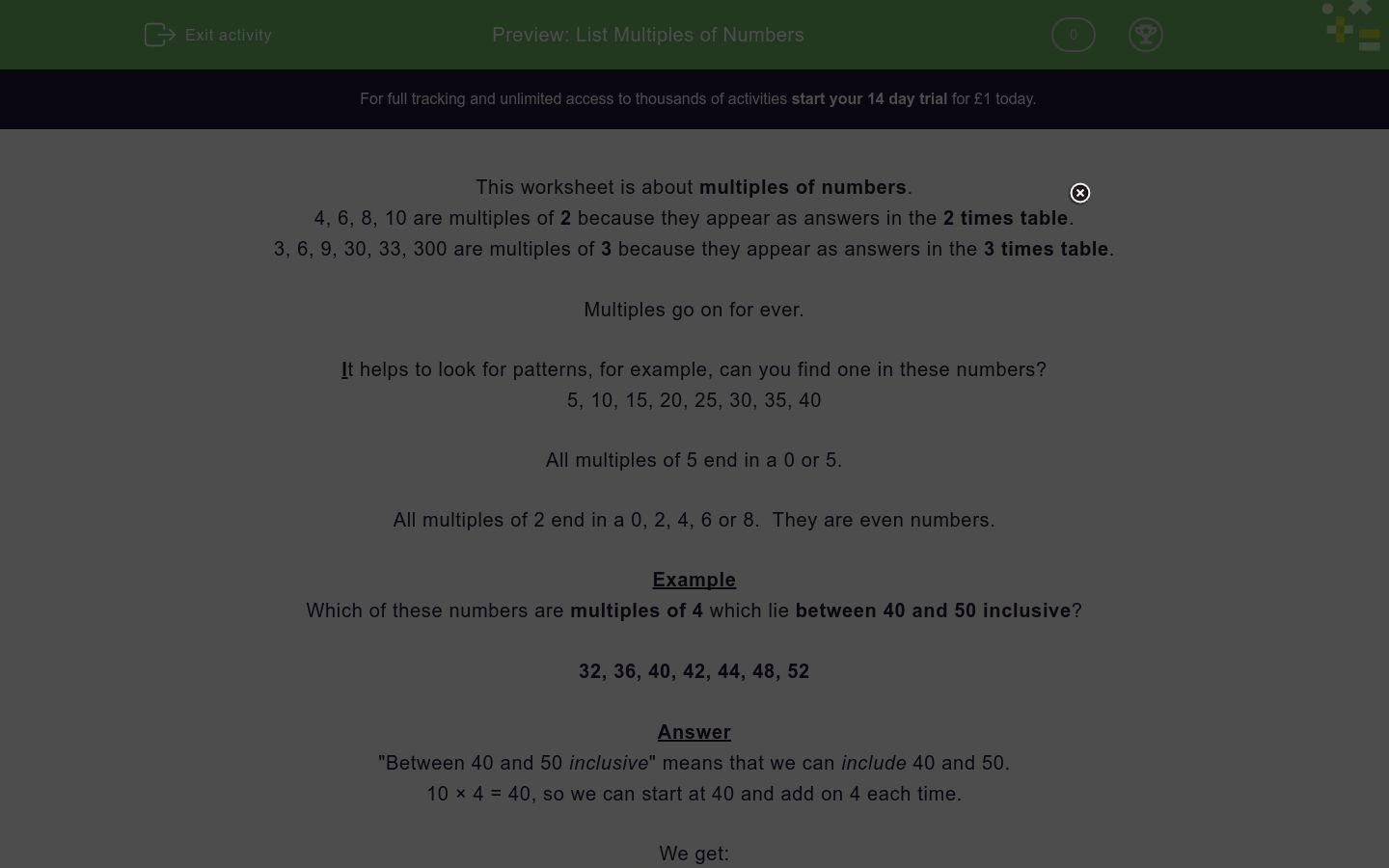# List Multiples of Numbers

In this worksheet, students list multiples of certain numbers between two limits.Key stage:  KS 2

Curriculum topic:   Number: Number and Place Value

Curriculum subtopic:   Count in Multiples (4, 8, 50 and 100)

Difficulty level:\$appendString = '&new=1'; \$_SESSION['guest_aId'] = rand(); \$urlToRedirect = FRAMEWORK_PATH . 'preview/worksheet/introduction?aId=' . \$_SESSION['guest_aId'] . '&wId=' . \$wsData['id'] . '&usertype=guest' . \$appendString; ?>

### QUESTION 1 of 10

This worksheet is about multiples of numbers.

4, 6, 8, 10 are multiples of 2 because they appear as answers in the 2 times table.

3, 6, 9, 30, 33, 300 are multiples of 3 because they appear as answers in the 3 times table.

Multiples go on for ever.

It helps to look for patterns, for example, can you find one in these numbers?

5, 10, 15, 20, 25, 30, 35, 40

All multiples of 5 end in a 0 or 5.

All multiples of 2 end in a 0, 2, 4, 6 or 8.  They are even numbers.

Example

Which of these numbers are multiples of 4 which lie between 40 and 50 inclusive?

32, 36, 40, 42, 44, 48, 52

"Between 40 and 50 inclusive" means that we can include 40 and 50.

10 × 4 = 40, so we can start at 40 and add on 4 each time.

We get:

40, 44, and 48.

Want to understand this further and learn how this links to other topics in maths?
Why not watch this video?

Select all the multiples of 2 which lie between 17 and 28.

18

20

22

23

24

26

Select all the multiples of 5 which lie between 66 and 81.

70

72

73

75

80

83

Select all the multiples of 5 which lie between 45 and 57.

50

51

53

55

56

57

Select all the multiples of 10 which lie between 20 and 54 inclusive.

20

25

30

35

40

50

Select all the multiples of 3 which are less than 15.

3

5

6

9

12

14

Select all the multiples of 6 which are less than 20.

2

3

6

12

18

20

Select all the multiples of 9 which are less than 30.

9

27

30

81

18

24

Select all the multiples of 5 which lie between 30 and 49 inclusive.

30

45

35

50

25

44

Select all the multiples of 4 which lie between 30 and 50 inclusive.

32

36

40

44

46

48

Select all the numbers which are multiples of 2 lying between 32 and 71.

34

36

38

40

42

43

44

46

48

51

52

54

57

58

60

62

64

65

69

70

• Question 1

Select all the multiples of 2 which lie between 17 and 28.

18
20
22
24
26
EDDIE SAYS
Multiples of 2 end in 0, 2, 4, 6 or 8.
The multiples of 2 between these two numbers are:
18, 20, 22, 24, 26.
• Question 2

Select all the multiples of 5 which lie between 66 and 81.

70
75
80
EDDIE SAYS
Multiples of 5 end in a 0 or 5.
The multiples of 5 between these two numbers are:
70, 75, 80.
• Question 3

Select all the multiples of 5 which lie between 45 and 57.

50
55
EDDIE SAYS
Multiples of 5 end in a 0 or 5.
The multiples of 5 between these two numbers are:
50, 55.
• Question 4

Select all the multiples of 10 which lie between 20 and 54 inclusive.

20
30
40
50
EDDIE SAYS
Multiple of 10 end in a 0.
Note the word "inclusive".
The multiples of 10 between these two numbers are:
20, 30, 40, 50.
• Question 5

Select all the multiples of 3 which are less than 15.

3
6
9
12
EDDIE SAYS
These are the answers in the 3 times table, which are less than 15.
They are 3, 6, 9 and 12.
• Question 6

Select all the multiples of 6 which are less than 20.

6
12
18
EDDIE SAYS
These are the answers in the 6 times table, which are less than 20.
They are 6, 12, and 18.
• Question 7

Select all the multiples of 9 which are less than 30.

9
27
18
EDDIE SAYS
These are the answers in the 9 times table, which are less than 30.
They are 9, 18, and 27.
• Question 8

Select all the multiples of 5 which lie between 30 and 49 inclusive.

30
45
35
EDDIE SAYS
Multiples of 5 end in 0 or 5.
Note the word "inclusive".
The multiples of 5 between these two numbers are:
30, 35
40, 45.
• Question 9

Select all the multiples of 4 which lie between 30 and 50 inclusive.

32
36
40
44
48
EDDIE SAYS
These are the answers to the 4 times table which lie between 30 and 50.
They are 32, 36, 40, 44 and 48.
• Question 10

Select all the numbers which are multiples of 2 lying between 32 and 71.

34
36
38
40
42
44
46
48
52
54
58
60
62
64
70
EDDIE SAYS
Multiples of 2 end in 0, 2, 4, 6 or 8.
The multiples of 2 between these two numbers are:
34, 36, 38,
40, 42, 44, 46, 48,
50, 52, 54, 56, 58,
60, 62, 64, 66, 68,
70.
---- OR ----

Sign up for a £1 trial so you can track and measure your child's progress on this activity.

### What is EdPlace?

We're your National Curriculum aligned online education content provider helping each child succeed in English, maths and science from year 1 to GCSE. With an EdPlace account you’ll be able to track and measure progress, helping each child achieve their best. We build confidence and attainment by personalising each child’s learning at a level that suits them.

Get started